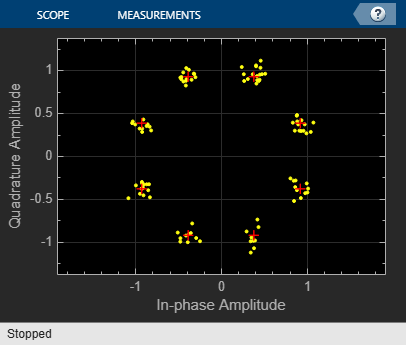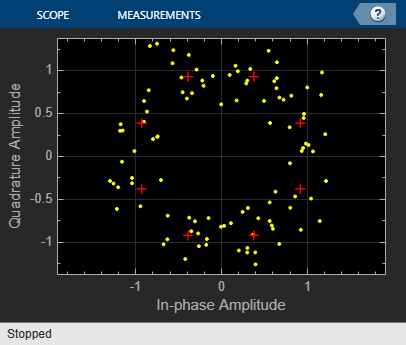# Modulate and Demodulate 8-PSK Signal

Open the 8-PSK model. The model generates an 8-PSK signal, applies white noise, displays the resulting constellation diagram, and computes the error statistics.Run the model.The error statistics are collected in vector `ErrorVec`. Because Eb/No is 15 dB, there are no measured symbol errors.

```Number of symbol errors = 0 ```

Change the Eb/No of the AWGN Channel block from 15 dB to 5 dB. The increase in the noise is shown in the constellation diagram.Because of the increase in the noise level, the number of symbol errors is greater than zero.

```Number of symbol errors = 13 ```